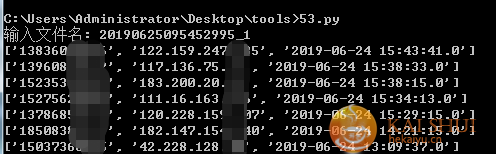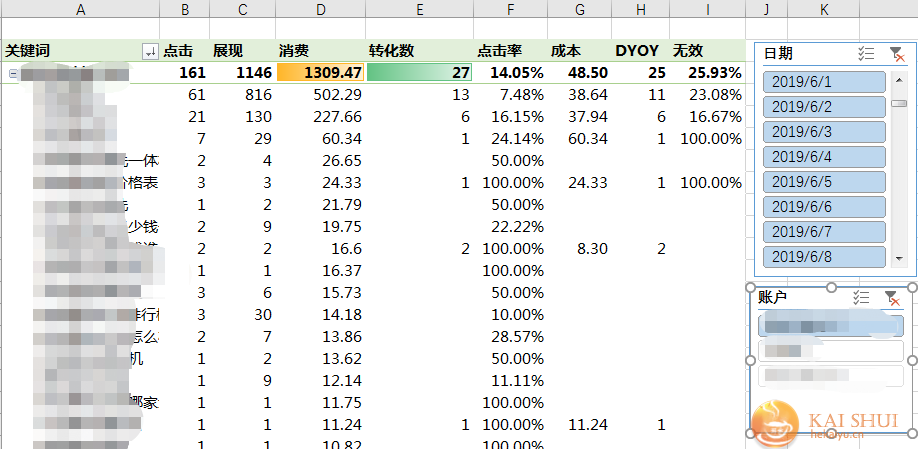• 业务合作 全媒体搜索+信息流，招商医疗保健品等行业竞价开户，请咨询客服QQ:40974387。
• 业务合作 SEM竞价托管，竞价单页制作，网站制作，请咨询客服QQ:40974387。

# 留言信息反查53KF详细数据，python与sem

## 背景

53客服可以根据聊天内容搜索到具体访客数据，但是只能一条一条来，更不用说开放什么api了。在做数据分析中除了要统计转换外，无效分析也是必须的，然而客服反馈回来的无效往往只有电话号码。这里更难受的又来了，53下载下来的数据只有关键词、咨询页面等信息，并不包含电话。。。所以无法直接vlookup。## python提取53关键信息

1. 下载53的html数据后，提取所有的ip、时间放入数组；
2. 然后整理出每个访客的对话内容，清除换行、空格等干扰信息；
3. 逐行读取无效电话内容，如果对话内容包含，则是无效，输出对应ip 时间，到数组；
4. 导出结果。```# -*- coding: utf-8 -*-
import pandas as pd
from bs4 import BeautifulSoup

path = input('输入文件名: ')
path = path + '.html'
with open(path, 'r',encoding='UTF-8') as f:
table=Soup.find('table').find_all('tr')
i=0
j=0
ips=[]
tms=[]
dhs=[]
jg=[]
for ttr in table:
try:
tds=ttr.find_all('td',class_="td_0")
ip=tds.get_text().replace('\n','').replace('\r','').replace('\r\n','')
time=tds.get_text().replace('\n','').replace('\r','').replace('\r\n','')
ips.append(ip)
tms.append(time)
i=i+1
except:
pass
worker_msgs=Soup.find_all('tr',class_="worker_msg")
dh=open("dh.txt", 'r',encoding='UTF-8')
l=list(line.split())
dhs.append(l)
for ttr in worker_msgs:
uu=ttr.get_text()
uu = uu.replace('\n','').replace('\r','').replace('\r\n','')
for dh in dhs:
if dh in uu:
l=[dh,ips[j],tms[j]]
jg.append(l)
print (l)
j=j+1
name=['info','ip','time']
test=pd.DataFrame(columns=name,data=jg)
print(test)
test.to_csv('ip_jmk_time.csv',encoding='UTF-8')
```

## vlookup使用方法

VLOOKUP函数是Excel中的一个纵向查找函数，功能是按列查找，最终返回该列所需查询序列所对应的值；与之对应的HLOOKUP是按行查找的。详细使用方法：

https://baike.baidu.com/item/VLOOKUP函数/

`=VLOOKUP(F925,I:K,3,0)`

## 无效分析

1、初级分析，通过vlookup函数利用ip将无效原因输出到53excel版本的数据中，满足基本的时间段或者是地域的无效分析。

2、加入数据模型，系统分析关键词、搜索词、单元、计划、时间、地域的无效情况。EXCEL竞价自动分析，Power Pivot数据建模分析

```= Table.AddColumn(重命名的列4, "是否无效", each if List.Contains(ip_jmk[ip],[ip]) then "是" else null)

List.Contains({1,2,3,4},3)
true```

List.Contains指示列表 list 中是否包含值value，返回逻辑值。手机阅读 | 转载注明：http://www.hekaiyu.cn/sem/4136.html